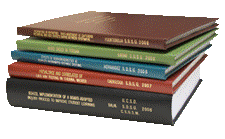Archival Master’s Theses from the University of Nebraska-Lincoln

Thesis

6-1957

Citation

Thesis (M.S.)—University of Nebraska—Lincoln, 1957. Department of Agricultural Engineering.

Copyright 1957, the author. Used by permission.

Abstract

Water application, storage, and distribution efficiencies of sprinkler and furrow methods of irrigation were compared at North Platte, Nebraska. Four plots of Midland grain sorghum were irrigated with portable sprinkler lines and four with gated pipe and furrows. Cultural practices were the same for all plots with the exception that the sprinkler plots did not receive any cultivation at the time of furrowing the plots to be furrow irrigated. The results obtained were:

1. Runoff losses on the sprinkler plots averaged 0.5 per cent of the water applied. On the furrow plots 6.67 per cent of applied water ran off.
2. Evaporation and deep percolation were assumed to be the difference between runoff loss and total water loss. These combined losses were 16.8 per cent of the water applied on sprinkler plots and 19.1 per cent of the applied water on furrow plots.
3. The water application efficiency on sprinkler plots averaged 82.7 per cent as compared to an average of 74.2 per cent on furrow irrigated plots.
4. The water storage efficiency on furrow irrigated plots averaged 93.0 per cent as compared to an average of 88.5 per cent on sprinkler plots.
5. Water distribution efficiencies provide a direct means of comparing areas within a plot or corresponding areas of different plots to determine uniformity of water application. The average distribution efficiency lengthwise of the plots varied from 8.2 percentage points on sprinkler plots as compared to 38.9 percentage points on furrow irrigated plots. The average distribution efficiency across the plots varied from 8.1 percentage points on furrow irrigated plots to 12.7 percentage points on sprinkler plots.
6. The average yield of grain sorghum was 69.1 bushels per acre for furrow irrigation. The average yield of grain sorghum was 67.6 bushels per acre for sprinkler irrigation. The difference in yield 1.5 bushels per acre was not significant at the 5 per cent level of probability.